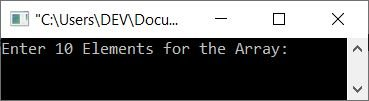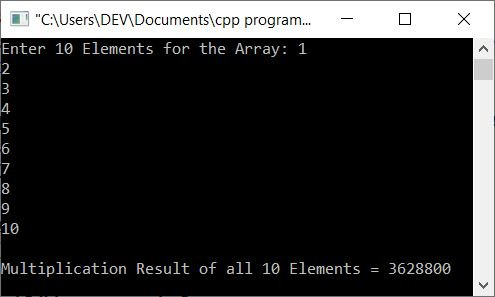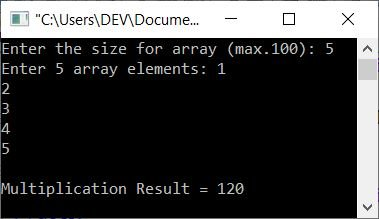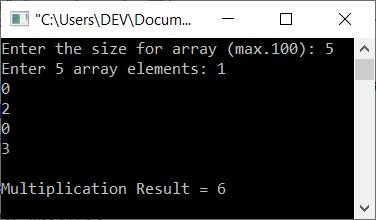# C++ program to multiply all elements (numbers) in an array

This article includes some programs in C++ that find and print the multiplication result of all elements in a given array. These are the lists of programs available here:

## Multiply all elements in an array of 10 elements

The question is, "Write a program in C++ that receives 10 elements for an array to find and print the multiplication result of all the given 10 elements." Here is its answer:

```#include<iostream>

using namespace std;
int main()
{
int arr, i, mul=1;
cout<<"Enter 10 Elements for the Array: ";
for(i=0; i<10; i++)
cin>>arr[i];
for(i=0; i<10; i++)
mul = mul*arr[i];
cout<<"\nMultiplication Result of all 10 Elements = "<<mul;
cout<<endl;
return 0;
}```

Here is the initial output produced by the above C++ program on printing the multiplication result of all elements in a given array:Now supply any 10 elements, say 1, 2, 3, 4, 5, 6, 7, 8, 9, 10 and press ENTER to find and print the multiplication result of all these elements as shown in the snapshot given below:## Find the multiplication result of all elements in an array of n elements

This program allows the user to define the size of the array along with its elements. That is, the program receives the size, then asks to enter all the elements for an array of the required size to multiply them, and then prints the multiplication result:

```#include<iostream>

using namespace std;
int main()
{
int n, arr, i, m=1;
cout<<"Enter the size for array (max.100): ";
cin>>n;
cout<<"Enter "<<n<<" array elements: ";
for(i=0; i<n; i++)
{
cin>>arr[i];
m *= arr[i];
}
cout<<"\nMultiplication Result = "<<m;
cout<<endl;
return 0;
}```

Here is a sample run with user input of 5 as the size or value of n and 1, 2, 3, 4, and 5 as the five numbers or array elements:## Find the multiplication of only non-zero array elements

Since both the programs given above give 0 as a result, if any of the elements in an array are 0, because multiplying any number with 0 gives 0 as a result. Therefore, let's modify the above program and create a new one that only finds and prints the multiplication result of non-zero elements:

```#include<iostream>

using namespace std;
int main()
{
int n, arr, i, m=1, chk=0;
cout<<"Enter the size for array (max.100): ";
cin>>n;
cout<<"Enter "<<n<<" array elements: ";
for(i=0; i<n; i++)
{
cin>>arr[i];
if(arr[i]!=0)
{
m *= arr[i];
chk = 1;
}
}
if(chk==1)
cout<<"\nMultiplication Result = "<<m;
else
cout<<endl;
return 0;
}```

Here is its sample run with user input of 5 as size and 1, 0, 2, 0, 3 as five array elements:C++ Quiz

« Previous Program Next Program »